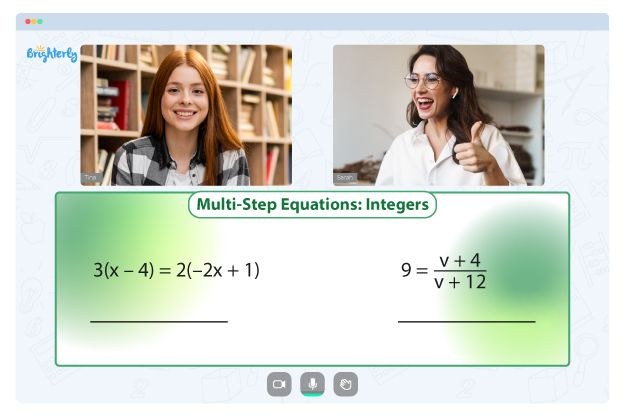Whole numbers, negative numbers, and zero are all examples of integers. Some kids still mistake fractions and decimals to be integers while doing their math, so it’s essential to help a kid understand the concept. Check out how a 7th grade integers worksheet can help youngsters learn better.

Brighterly’s tutors use 7th grade integers worksheet grade 7 to help students understand how integers work. Instructors use an integers worksheet grade 7 PDF to introduce students to every computation involving positive or negative numbers.

Math for Kids

Is Your Child Struggling With Math?
1:1 Online Math TutoringUsing subtracting integers worksheet grade 7, students can better grasp numbers in a shorter period. Finally, students can get acquainted with an integers worksheet grade 7 and excel in their schoolwork.

## How to Use 7th Grade Integers Worksheets in a Fun Way### 7th Grade Integers Worksheets PDF### 7th Grade Integers Worksheets PDF### 7th Grade Integers Worksheets PDF### 7th Grade Integers Worksheets PDF

Students will consider integer worksheets grade 7 as a fun alternative to textbooks since they see colorful images and do exciting activities. Before long, students will get acquainted with an adding and subtracting integers worksheet 7th grade and have a better shot at learning.

Troubles with Equations?• Is your child finding it challenging to understand of equations?
• An online tutor could be the solution.

Does your child struggle to master equations? Try lessons with an online tutor.